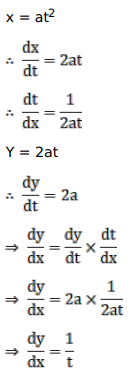# Solve this following

Question:

Mark $(\sqrt{)})$ against the correct answer in the following:

If $x=a t^{2}, y=2 a t$ then $\frac{d y}{d x}=?$

A. $\frac{1}{t}$

B. $\frac{-1}{t^{2}}$

C. $\frac{-2}{t}$

D. none of these

Solution: